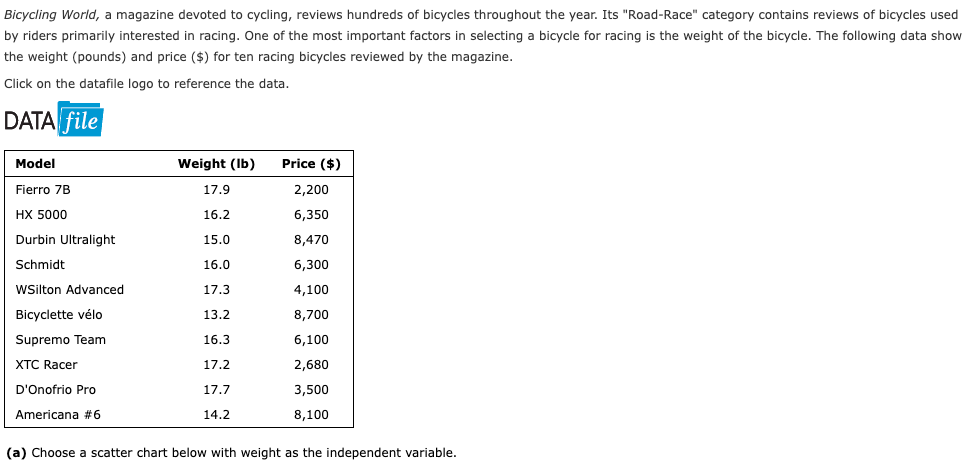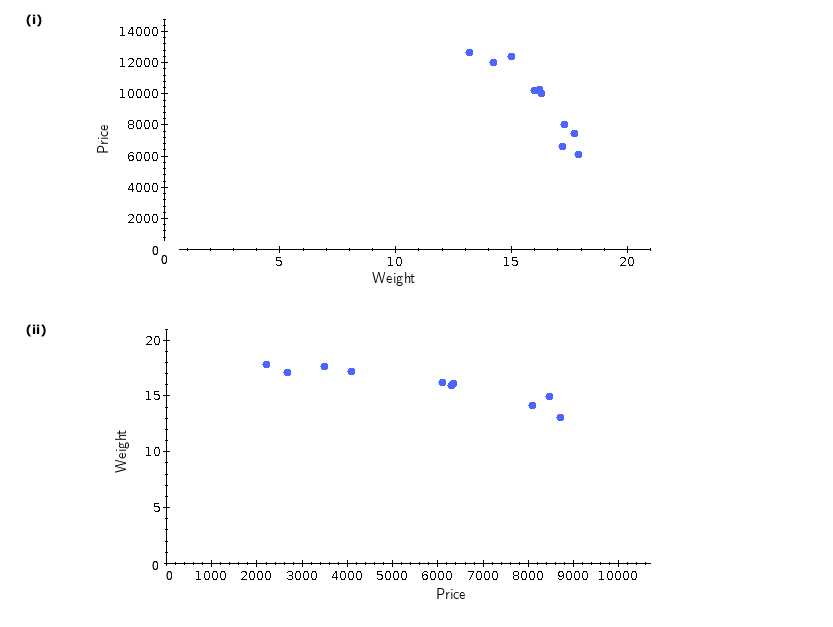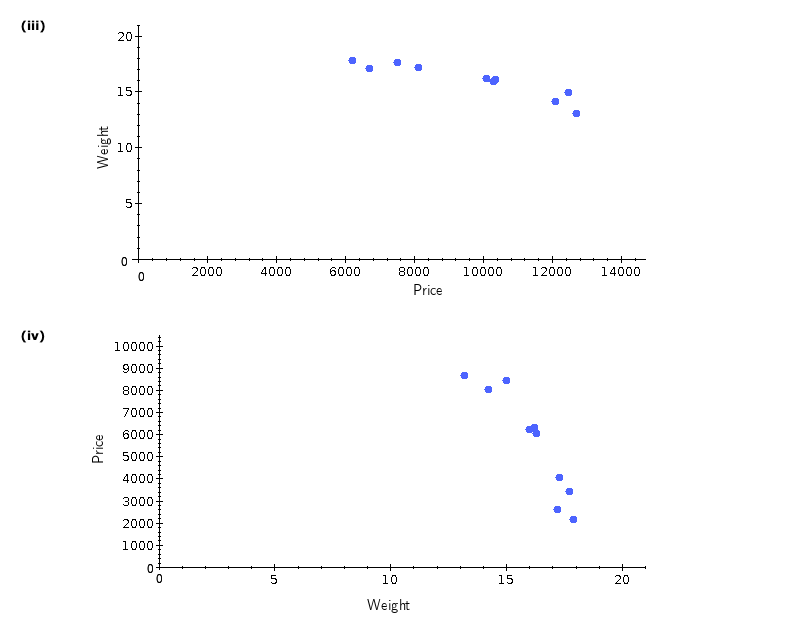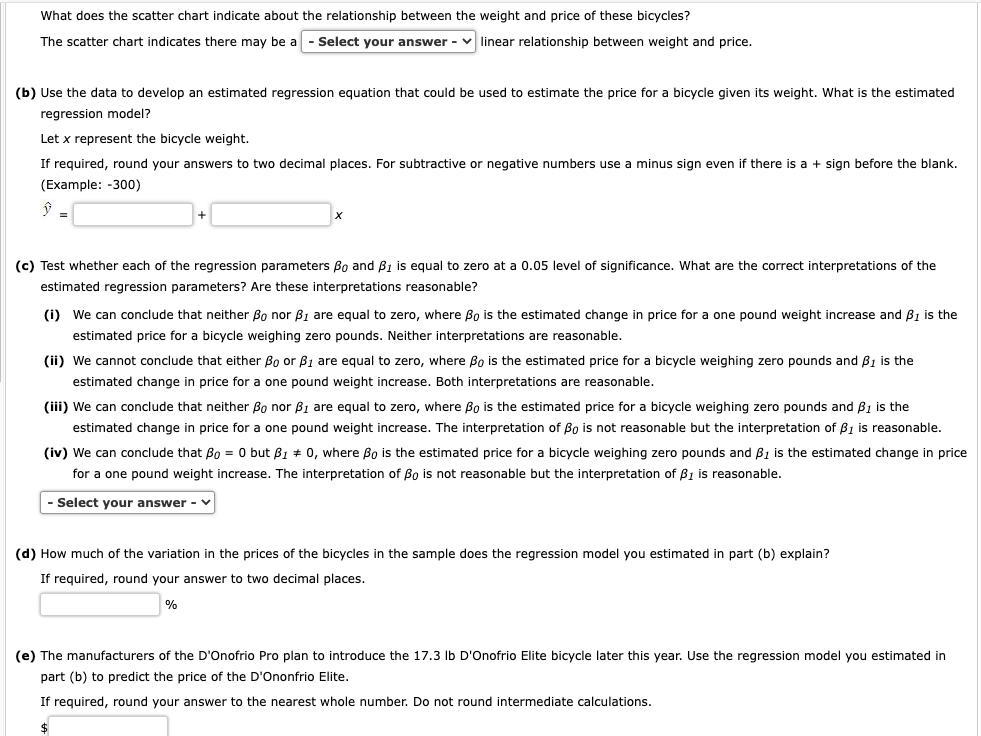Home / Expert Answers / Statistics and Probability / bicycling-world-a-magazine-devoted-to-cycling-reviews-hundreds-of-bicycles-throughout-the-year-pa425

# (Solved): Bicycling World, a magazine devoted to cycling, reviews hundreds of bicycles throughout the year. ...Bicycling World, a magazine devoted to cycling, reviews hundreds of bicycles throughout the year. Its "Road-Race" category contains reviews of bicycles used by riders primarily interested in racing. One of the most important factors in selecting a bicycle for racing is the weight of the bicycle. The following data show the weight (pounds) and price (\\$) for ten racing bicycles reviewed by the magazine. Click on the datafile logo to refere the data. DATA (a) Choose a scatter chart below with weight as the independent variable. (ii) (iii) (b) Use the data to develop an estimated regression equation that could be used to estimate the price for a bicycle given its weight. What is the estimated regression model? Let represent the bicycle weight. If required, round your answers to two decimal places. For subtractive or negative numbers use a minus sign even if there is a + sign before the blank. (Example: -300) (c) Test whether each of the regression parameters and is equal to zero at a 0.05 level of significance. What are the correct interpretations of the estimated regression parameters? Are these interpretations reasonable? (i) We can conclude that neither nor are equal to zero, where is the estimated change in price for a one pound weight increase and is the estimated price for a bicycle weighing zero pounds. Neither interpretations are reasonable. (ii) We cannot conclude that either or are equal to zero, where is the estimated price for a bicycle weighing zero pounds and is the estimated change in price for a one pound weight increase. Both interpretations are reasonable. (iii) We can conclude that neither nor are equal to zero, where is the estimated price for a bicycle weighing zero pounds and is the estimated change in price for a one pound weight increase. The interpretation of is not reasonable but the interpretation is reasonable (iv) We can conclude that but , where is the estimated price for a bicycle weighing zero pounds and is the estimated change in price for a one pound weight increase. The interpretation of is not reasonable but the interpretation of is reasonable. (d) How much of the variation in the prices of the bicycles in the sample does the regression model you estimated in part (b) explain? If required, round your answer to two decimal places. (e) The manufacturers of the D'Onofrio Pro plan to introduce the D'Onofrio Elite bicycle later this year. Use the regression model you estimated in part (b) to predict the price of the D'Ononfrio Elite. If required, round your answer to the nearest whole number. Do not round intermediate calculations.

We have an Answer from Expert